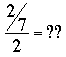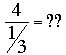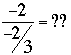SEARCH HOMEMath Central Quandaries & QueriesQuestion from Mitch, a student: -2 divided by -2/3 = ??Hi Mitch,

Let's work up to this.

10/2 = ?? That's easy, the answer is 5 since 2 × 5 = 10. This answer makes me think that I can ask the question in a different way. Instead of 10/2 = ?? I am going to ask What do you multiply the denominator by to get the numerator? Said in symbols 2 × ?? = 10.

Try anotherAgain I want to ask What do you multiply the denominator by to get the numerator? or
2 × ?? = 2/7 . Now you can see ?? = 1/7

And one moreReworded it becomes 1/3 × ?? = 4 and hence ?? = 12.

FinallyPennyMath Central is supported by the University of Regina and The Pacific Institute for the Mathematical Sciences.